Function Repository Resource:

# InverseBoole

Inverse of the Boole function

Contributed by: Daniel Sanchez (Wolfram Research)
 ResourceFunction["InverseBoole"][expr] yields True if expr is 1 and False if it is 0.

## Details and Options

ResourceFunction["InverseBoole"][expr] remains unchanged if expr is neither 1 nor 0.
ResourceFunction["InverseBoole"][expr] is effectively equivalent to Switch[expr,1,True,0,False].
ResourceFunction["InverseBoole"] is Listable.

## Examples

### Basic Examples (2)

 In:=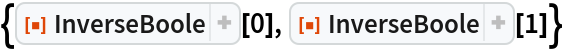Out=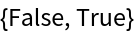Convert a List of 1's and 0's to truth values:

 In:=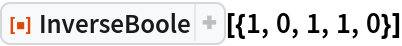Out=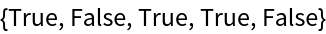### Applications (1)

InverseBoole is particularly useful when testing a condition based on bit values:

 In:=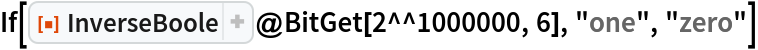Out=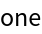### Properties and Relations (2)

InverseBoole is the inverse function of Boole:

 In:=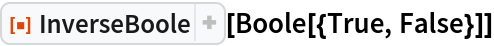Out=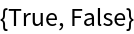InverseBoole deals with Rule just like Boole:

 In:=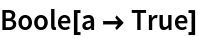Out=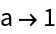In:=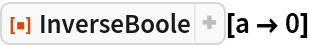Out=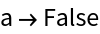### Possible Issues (3)

Only one argument is expected:

 In:=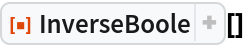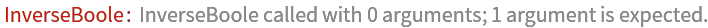Out=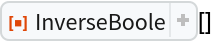In:=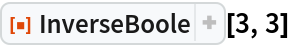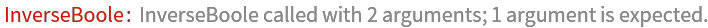Out=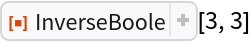If the sole argument provided is neither 1 nor 0, the expression is not evaluated:

 In:=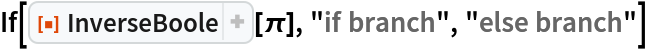Out=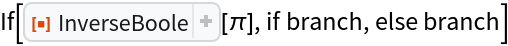If the argument is a numeric value, use Unitize together with InverseBoole:

 In:=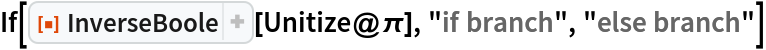Out=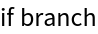Daniel Sanchez

## Version History

• 1.0.0 – 24 August 2020